﻿ PipeLay > Software Components & Operation > Defining a Vessel > Vessel Subcomponents > Support > Support Data Inputs

# Support Data Inputs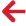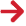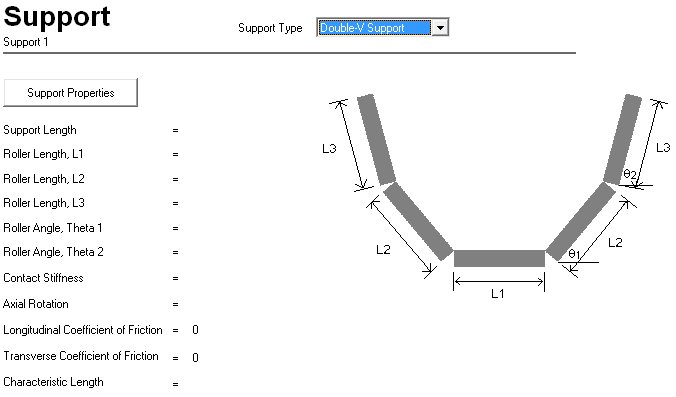Input Description Support Type: This drop-down list is used to select the type of support. The options are Double-V Support (the default), O-Shaped Support and Zero-Gap O Support. Support Properties Click on this button to display a dialog that allows you to enter the properties of the support. This dialog varies depending on the type of support that you select from the Support Type drop-down list.  See the ‘Double-V Support Data Dialog’, ‘O-Shaped Roller Data Dialog’ and ‘Zero-gap Guide Properties Dialog’ sections in this article for more information.

## Double-V Support Data Dialog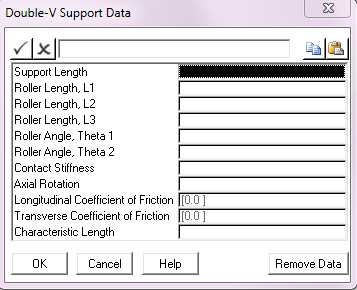Input Description Support Length: The length of the support in the axial direction. Units: [m] or [ft] Roller Length, L1: The length of first contact surface. See Note (a) below. Units: [m] or [ft] Roller Length, L2: The length of the second contact surface. Units: [m] or [ft] Roller Length, L3: The length of the third contact surface.  See Note (a) below. Units: [m] or [ft] Roller Angle, Theta 1: The angle of the second contact surface. See Note (a) below. Units: [degrees] Roller Angle, Theta 2: The angle of the third contact surface. See Note (a) below. Units: [degrees] Contact Stiffness: The contact stiffness of the support. This is an optional input and if omitted then a suitable default optimum stiffness is calculated during subsequent analyses. The relevant calculation procedure is discussed in Technical Note 5. Units: [kN/m] or [kips/ft] Axial Rotation: The angle of rotation about the support axis. The Axial Rotation allows you to rotate the rollerbox about its centre point. This centre point (or centre of rotation) is located half-way along the lowest surface of the support (in general, at 0.5 * L1 in the figure below). The figure below shows the effect of rotation on a typical rollerbox. No rotation is applied by default. Units: [degrees] Longitudinal Coefficient of Friction: The coefficient of friction between the structure and the support in the longitudinal or axial direction. This defaults to zero. Transverse Coefficient of Friction: The coefficient of friction between the structure and the support in the transverse direction. This defaults to zero. Characteristic Length: The length to be used in determining the non-linear stiffness used to simulate frictional restraint on the support. This input is optional. The Characteristic Length input relates to the operation of the friction model, and allows user control over the characteristics of non-linear springs used to model frictional restraint. This input is optional, and defaults to 10% of the support length. Modelling of friction is described in conjunction with the various contact modelling options in Technical Note 5. Units: [m] or [ft]

Notes:

 (a) The geometry of a Double–V rollerbox support is defined in terms of a series of lengths and angles as shown in the 'Double-V Support' figure below.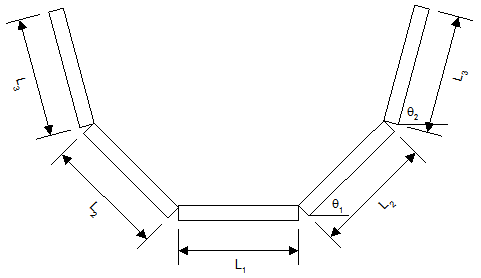Double-V Support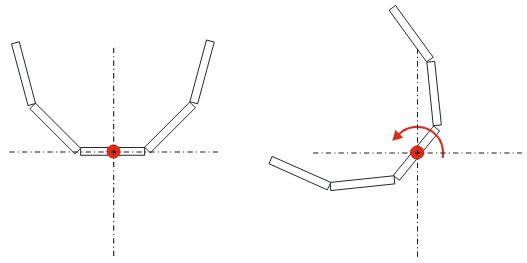Axial Rotation

## O-Shaped Roller Data Dialog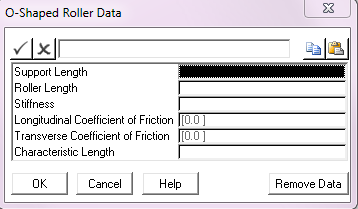Input Description Support Length: The length of the O-shaped support in the axial direction. PipeLay models O-shaped roller boxes using an equivalent “double square” approximation. Four flat surfaces are combined to create a square enclosure and a second identical box, oriented 45° to the first, is combined with it. This support type is effectively superseded by the more general Double-V support described earlier. Units: [m] or [ft] Roller Length: The roller length of the O-shaped support. This is equal to the side of the square in the “double square” approximation. See the description above for Support Length for more information. Units: [m] or [ft] Stiffness: The contact stiffness of the support. This is an optional input and if omitted then a suitable default optimum stiffness is calculated during subsequent analyses. The relevant calculation procedure is discussed in Units: [kN/m] or [kips/ft] Longitudinal Coefficient of Friction: The coefficient of friction between the structure and the support in the longitudinal or axial direction. This defaults to zero. Transverse Coefficient of Friction: The coefficient of friction between the structure and the support in the transverse direction. This defaults to zero. Characteristic Length: The length to be used in determining the non-linear stiffness used to simulate frictional restraint on the support. This input is optional. The Characteristic Length input relates to the operation of the friction model, and allows user control over the characteristics of non-linear springs used to model frictional restraint. This input is optional, and defaults to 10% of the support length. Modelling of friction is described in conjunction with the various contact modelling options in Technical Note 5. Units: [m] or [ft]

## Zero-gap Guide Properties Dialog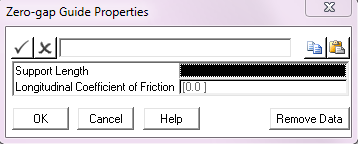Input Description Support Length: The length of the zero-gap O support in the axial direction. Units: [m] or [ft] Longitudinal Coefficient of Friction: The coefficient of friction between the structure and the support in the longitudinal or axial direction. This defaults to zero.